## Adding and Subtracting Rational Expressions

To add or subtract rational expressions with the same denominators:

• Add or subtract the numerators as indicated.

• Keep the common denominator.

• Simplify the resulting rational expression if possible.

##### Example 1

Simplify.##### Example 2

Simplify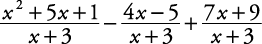.To add or subtract rational expressions with different denominators:

1. Completely factor each denominator.

2. Find the least common denominator (LCD) for all the denominators by multiplying together the different prime factors with the greatest exponent for each factor.

3. Rewrite each fraction so it has the LCD as its denominator by multiplying each fraction by the value 1 in an appropriate form.

4. Combine numerators as indicated and keep the LCD as the denominator.

5. Simplify the resulting rational expression if possible.

##### Example 3

Simplify.

Completely factor each denominator.

• x and y are already prime factors.

Find the least common denominator (LCD) for all the denominators.

• The LCD = xy.

Rewrite each fraction so it has the LCD as its denominator.Combine numerators and keep the LCD as the denominator.This rational expression cannot be simplified further. Therefore,##### Example 4

Simplify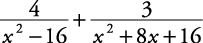.

Factor each denominator.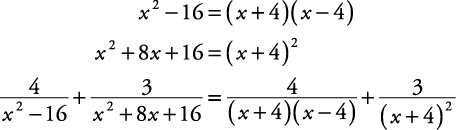Find the LCD.

The LCD = ( x – 4)( x + 4) 2

Rewrite each fraction so that the LCD is its denominator.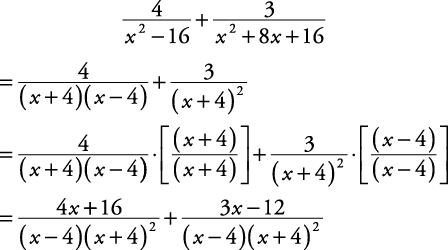Combine numerators and keep the LCD as the denominator.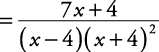This rational expression cannot be simplified further. Therefore,##### Example 5

Simplify.

( x – 3) is a prime factor.

Rewrite in descending order.

9 – x 2 = – x 2 + 9

Factor out –1 so the leading coefficient is positive.The LCD = ( x – 3)( x + 3). [The LCD could also have been –1( x – 3)( x + 3).]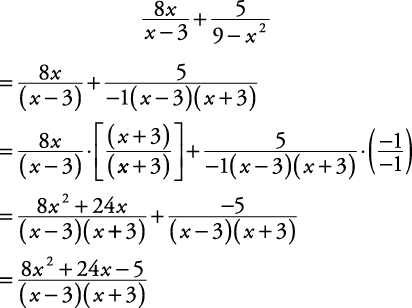This rational expression cannot be simplified further. Therefore,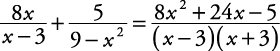##### Example 6

Simplify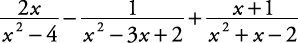.

Factor each denominator.The LCD = ( x + 2)( x – 2)( x – 1).

Rewrite the fraction so the LCD is the denominator.The LCD is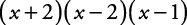This rational expression can be simplified.Therefore,